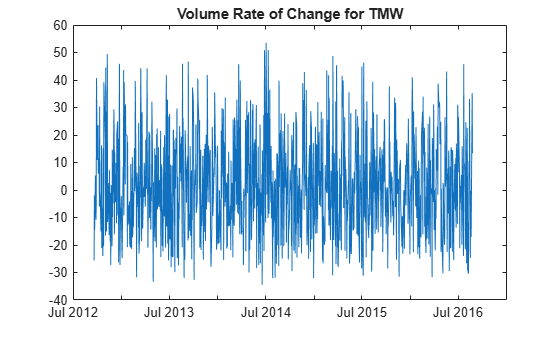# volroc

Volume rate of change

Using a `fints` object for the `Data` argument of `volroc` is not recommended. Use a vector, `timetable`, or `table` instead for financial time series. For more information, see Convert Financial Time Series Objects fints to Timetables.

## Syntax

``volumeChangeRate = volroc(Data)``
``volumeChangeRate = volroc(___,Name,Value)``

## Description

example

````volumeChangeRate = volroc(Data)` calculates the volume rate-of-change from a data series of volume traded. The volume rate-of-change is calculated between the current volume and the volume n periods ago. By default, the Volume rate of change is based on a 12-period difference.```

example

````volumeChangeRate = volroc(___,Name,Value)` adds optional name-value pair arguments. ```

## Examples

collapse all

Load the file `SimulatedStock.mat`, which provides a timetable (`TMW`) for financial data for TMW stock.

```load SimulatedStock.mat volumeChangeRate = volroc(TMW); plot(volumeChangeRate.Time,volumeChangeRate.VolumeChangeRate) title('Volume Rate of Change for TMW')```## Input Arguments

collapse all

Data for volume traded, specified as a vector, table, or timetable. For vector input, `Data` is an `M`-by-`1` column vector of volume traded. Timetables and tables with `M` rows must contain a variable named `'Volume'` (case insensitive).

Data Types: `double` | `table` | `timetable`

### Name-Value Pair Arguments

Specify optional comma-separated pairs of `Name,Value` arguments. `Name` is the argument name and `Value` is the corresponding value. `Name` must appear inside quotes. You can specify several name and value pair arguments in any order as `Name1,Value1,...,NameN,ValueN`.

Example: ```volumeChangeRate = volroc(TMW,'NumPeriods',18)```

Period difference for `volumeChangeRate`, specified as the comma-separated pair consisting of `'NumPeriods'` and a scalar positive integer.

Data Types: `double`

## Output Arguments

collapse all

Volume rate of change series, returned with the same number of rows (`M`) and the same type (vector, table, or timetable) as the input `Data`.

 Achelis, S. B. Technical Analysis from A to Z. Second Edition. McGraw-Hill, 1995, pp. 310–311.